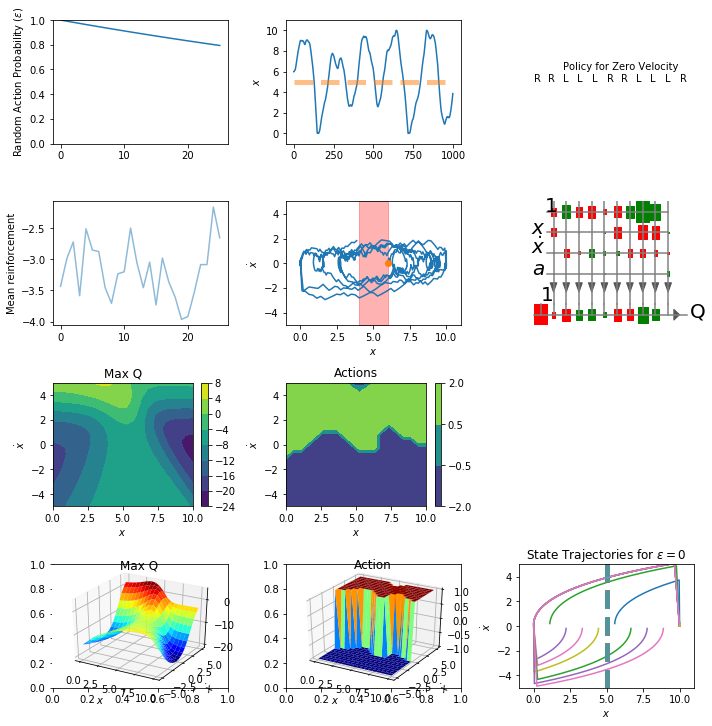$$\newcommand{\Rv}{\mathbf{R}} \newcommand{\rv}{\mathbf{r}} \newcommand{\Qv}{\mathbf{Q}} \newcommand{\Qnv}{\mathbf{Qn}} \newcommand{\Av}{\mathbf{A}} \newcommand{\Aiv}{\mathbf{Ai}} \newcommand{\av}{\mathbf{a}} \newcommand{\xv}{\mathbf{x}} \newcommand{\Xv}{\mathbf{X}} \newcommand{\yv}{\mathbf{y}} \newcommand{\Yv}{\mathbf{Y}} \newcommand{\zv}{\mathbf{z}} \newcommand{\av}{\mathbf{a}} \newcommand{\Wv}{\mathbf{W}} \newcommand{\wv}{\mathbf{w}} \newcommand{\betav}{\mathbf{\beta}} \newcommand{\gv}{\mathbf{g}} \newcommand{\Hv}{\mathbf{H}} \newcommand{\dv}{\mathbf{d}} \newcommand{\Vv}{\mathbf{V}} \newcommand{\vv}{\mathbf{v}} \newcommand{\Uv}{\mathbf{U}} \newcommand{\uv}{\mathbf{u}} \newcommand{\tv}{\mathbf{t}} \newcommand{\Tv}{\mathbf{T}} \newcommand{\TDv}{\mathbf{TD}} \newcommand{\Tiv}{\mathbf{Ti}} \newcommand{\Sv}{\mathbf{S}} \newcommand{\Gv}{\mathbf{G}} \newcommand{\zv}{\mathbf{z}} \newcommand{\Zv}{\mathbf{Z}} \newcommand{\Norm}{\mathcal{N}} \newcommand{\muv}{\boldsymbol{\mu}} \newcommand{\sigmav}{\boldsymbol{\sigma}} \newcommand{\phiv}{\boldsymbol{\phi}} \newcommand{\Phiv}{\boldsymbol{\Phi}} \newcommand{\Sigmav}{\boldsymbol{\Sigma}} \newcommand{\Lambdav}{\boldsymbol{\Lambda}} \newcommand{\half}{\frac{1}{2}} \newcommand{\argmax}{\underset{#1}{\operatorname{argmax}}} \newcommand{\argmin}{\underset{#1}{\operatorname{argmin}}} \newcommand{\dimensionbar}{\underset{#1}{\operatorname{|}}} \newcommand{\grad}{\mathbf{\nabla}} \newcommand{\ebx}{e^{\betav_{#1}^T \xv_n}} \newcommand{\eby}{e^{y_{n,#1}}} \newcommand{\Tiv}{\mathbf{Ti}} \newcommand{\Fv}{\mathbf{F}} \newcommand{\ones}{\mathbf{1}_{#1}}$$

# Reinforcement Learning to Control a Marble¶

Remember the one-dimensional maze example from last time.

We can make our one-dimensional maze problem into a realistic control problem by saying we have a marble on a one-dimensional track that has a mass and real-valued position and velocity values. So the state is actually two-dimensional, position, $x$, and velocity, $\dot{x}$. Actions are forces on the marble and let's just allow three forces, $-1$, $0$, and $1$.

In :
import sys
sys.path = ['.'] + sys.path

In :
import numpy as np
import matplotlib.pyplot as plt
%matplotlib inline
import neuralnetworks as nn   # from notes 09


As before, we define the reinforcement, initialState, and nextState functions to specify our dynamic marble problem.

In :
from copy import copy

actions = (-1, 0, 1)   # Possible actions

def reinforcement(s,s1):
goal = 5
# return 0 if abs(s1-goal) < 1 else -1
return -abs(s1 - goal)

def initialState():
return np.array([10*np.random.random_sample(), 0.0])

def nextState(s,a):
s = copy(s)   # s is position, s is velocity. a is -1, 0 or 1
deltaT = 0.1                           # Euler integration time step
s += deltaT * s                  # Update position
s += deltaT * (2 * a - 0.2 * s)  # Update velocity. Includes friction
if s < 0:        # Bound next position. If at limits, set velocity to 0.
s = [0,0]
elif s > 10:
s = [10,0]
return s


We also need our epsilonGreedy function.

In :
def epsilonGreedy(nnetQ, state, actions, epsilon):
if np.random.uniform() < epsilon:
# Random Move
action = np.random.choice(actions)
else:
# Greedy Move
Qs = [nnetQ.use(np.hstack((state,a)).reshape((1, -1))) for a in actions]
ai = np.argmax(Qs)
action = actions[ai]
Q = nnetQ.use(np.hstack((state,action)).reshape((1, -1)))
return action, Q


The makeSamples function is still general. We modify the one from the previous notes just a bit to allow our state variable to contain multiple values.

In :
def makeSamples(nnet, initialStateF, nextStateF, reinforcementF,
validActions, numSamples, epsilon):

X = np.zeros((numSamples, nnet.ni))
R = np.zeros((numSamples, 1))
Qn = np.zeros((numSamples, 1))

s = initialStateF()
s = nextStateF(s, 0)        # Update state, sn from s and a
a, _ = epsilonGreedy(nnet, s, validActions, epsilon)

# Collect data from numSamples steps
for step in range(numSamples):
sn = nextStateF(s, a)        # Update state, sn from s and a
rn = reinforcementF(s, sn)   # Calculate resulting reinforcement
an, qn = epsilonGreedy(nnet, sn, validActions, epsilon) # Forward pass for time t+1
X[step, :] = np.hstack((s,a))
R[step, 0] = rn
Qn[step, 0] = qn
# Advance one time step
s, a = sn, an

return (X, R, Qn)


Like before, let's make a plotStatus function to show us how we are doing.

In :
from mpl_toolkits.mplot3d import Axes3D
from matplotlib import cm

def plotStatus(net,trial,epsilonTrace,rtrace):
plt.subplot(4,3,1)
plt.plot(epsilonTrace[:trial+1])
plt.ylabel("Random Action Probability ($\epsilon$)")
plt.ylim(0,1)
plt.subplot(4,3,2)
plt.plot(X[:,0])
plt.plot([0,X.shape], [5,5],'--',alpha=0.5,lw=5)
plt.ylabel("$x$")
plt.ylim(-1,11)
#qs = [[net.use([s,0,a]) for a in actions] for s in range(11)]
qs = net.use(np.array([[s,0,a] for a in actions for s in range(11)]))
#print np.hstack((qs,-1+np.argmax(qs,axis=1).reshape((-1,1))))
plt.subplot(4,3,3)
acts = ["L","0","R"]
actsiByState = np.argmax(qs.reshape((len(actions),-1)),axis=0)
for i in range(11):
plt.text(i,0,acts[actsiByState[i]])
plt.xlim(-1,11)
plt.ylim(-1,1)
plt.text(2,0.2,"Policy for Zero Velocity")
plt.axis("off")
plt.subplot(4,3,4)
plt.plot(rtrace[:trial+1],alpha=0.5)
#plt.plot(np.convolve(rtrace[:trial+1],np.array([0.02]*50),mode='valid'))
binSize = 20
if trial+1 > binSize:
# Calculate mean of every bin of binSize reinforcement values
smoothed = np.mean(rtrace[:int(trial/binSize)*binSize].reshape((int(trial/binSize),binSize)),axis=1)
plt.plot(np.arange(1,1+int(trial/binSize))*binSize,smoothed)
plt.ylabel("Mean reinforcement")
plt.subplot(4,3,5)
plt.plot(X[:,0],X[:,1])
plt.plot(X[0,0],X[0,1],'o')
plt.xlabel("$x$")
plt.ylabel("$\dot{x}$")
plt.fill_between([4,6],[-5,-5],[5,5],color="red",alpha=0.3)
plt.xlim(-1,11)
plt.ylim(-5,5)
plt.subplot(4,3,6)
net.draw(["$x$","$\dot{x}$","$a$"],["Q"])

plt.subplot(4,3,7)
n = 20
positions = np.linspace(0,10,n)
velocities =  np.linspace(-5,5,n)
xs,ys = np.meshgrid(positions,velocities)
#states = np.vstack((xs.flat,ys.flat)).T
#qs = [net.use(np.hstack((states,np.ones((states.shape,1))*act))) for act in actions]
xsflat = xs.flat
ysflat = ys.flat
qs = net.use(np.array([[xsflat[i],ysflat[i],a] for a in actions for i in range(len(xsflat))]))
#qs = np.array(qs).squeeze().T
qs = qs.reshape((len(actions),-1)).T
qsmax = np.max(qs,axis=1).reshape(xs.shape)
cs = plt.contourf(xs,ys,qsmax)
plt.colorbar(cs)
plt.xlabel("$x$")
plt.ylabel("$\dot{x}$")
plt.title("Max Q")
plt.subplot(4,3,8)
acts = np.array(actions)[np.argmax(qs,axis=1)].reshape(xs.shape)
cs = plt.contourf(xs,ys,acts,[-2, -0.5, 0.5, 2])
plt.colorbar(cs)
plt.xlabel("$x$")
plt.ylabel("$\dot{x}$")
plt.title("Actions")

s = plt.subplot(4,3,10)
rect = s.get_position()
ax = Axes3D(plt.gcf(),rect=rect)
ax.plot_surface(xs,ys,qsmax,cstride=1,rstride=1,cmap=cm.jet,linewidth=0)
ax.set_xlabel("$x$")
ax.set_ylabel("$\dot{x}$")
#ax.set_zlabel("Max Q")
plt.title("Max Q")

s = plt.subplot(4,3,11)
rect = s.get_position()
ax = Axes3D(plt.gcf(),rect=rect)
ax.plot_surface(xs,ys,acts,cstride=1,rstride=1,cmap=cm.jet,linewidth=0)
ax.set_xlabel("$x$")
ax.set_ylabel("$\dot{x}$")
#ax.set_zlabel("Action")
plt.title("Action")

plt.tight_layout()


And, let's write a function to plop the marble down at a number of positions and try to control it, plotting the results.

In :
def testIt(Qnet,nTrials,nStepsPerTrial):
xs = np.linspace(0,10,nTrials)
plt.subplot(4,3,12)
for x in xs:
s = [x,0] ## 0 velocity
xtrace = np.zeros((nStepsPerTrial,2))
for step in range(nStepsPerTrial):
a,_ = epsilonGreedy(Qnet,s,actions,0.0) # epsilon = 0
s = nextState(s,a)
xtrace[step,:] = s
plt.plot(xtrace[:,0],xtrace[:,1])
plt.xlim(-1,11)
plt.ylim(-5,5)
plt.plot([5,5],[-5,5],'--',alpha=0.5,lw=5)
plt.ylabel('$\dot{x}$')
plt.xlabel('$x$')
plt.title('State Trajectories for $\epsilon=0$')

In :
def setupStandardization(net, Xmeans, Xstds, Tmeans, Tstds):
net.Xmeans = Xmeans
net.XstdsFixed = Xstds
net.Xconstant = [False] * len(Xmeans)
net.TstdsFixed = net.Tstds = Tstds
net.Tmeans = Tmeans
net.Tconstant = [False] * len(Tstds)

In [ ]:
from IPython.display import display, clear_output
fig = plt.figure(figsize=(10,10))

gamma = 0.8        # discount factor
nTrials = 500         # number of repetitions of makeSamples-updateQ loop
nStepsPerTrial = 1000 # number of steps between new random initial states
nSCGIterations = 4  # maximum number of SCG iterations
finalEpsilon = 0.01 # value of epsilon at end of simulation. Decay rate is calculated
epsilonDecay =  np.exp(np.log(finalEpsilon)/(nTrials)) # to produce this final value
print("epsilonDecay is",epsilonDecay)

nh = 10           # number of hidden units
nnetQ = nn.NeuralNetwork(3, nh, 1)  # ((0,10),(-3,3),(-1,1)))
# Inputs are position (1 to 10) velocity (-3 to 3) and action (-1, 0, or 1)
setupStandardization(nnetQ, [5, 0, 0], [2, 2, 0.5], , )

epsilon = 1         # initial epsilon value
epsilonTrace = np.zeros(nTrials)
rtrace = np.zeros(nTrials)
for trial in range(nTrials):
# Collect nStepsPerRep samples of X, R, Qn, and Q, and update epsilon

X, R, Qn = makeSamples(nnetQ,  initialState, nextState, reinforcement, actions, nStepsPerTrial, epsilon)
nnetQ.train(X, R + gamma*Qn, nIterations=nSCGIterations)

# X,R,Qn,Q,epsilon = getSamples(Qnet,actions,nStepsPerTrial,epsilon)
# Rest is for plotting
epsilonTrace[trial] = epsilon
epsilon *= epsilonDecay
rtrace[trial] = np.mean(R)
if True and (trial+1 == nTrials or trial % (nTrials/40) == 0):
fig.clf()
plotStatus(nnetQ, trial, epsilonTrace, rtrace)
testIt(nnetQ, 10, 500)
clear_output(wait=True)
display(fig)

clear_output(wait=True)In [ ]: